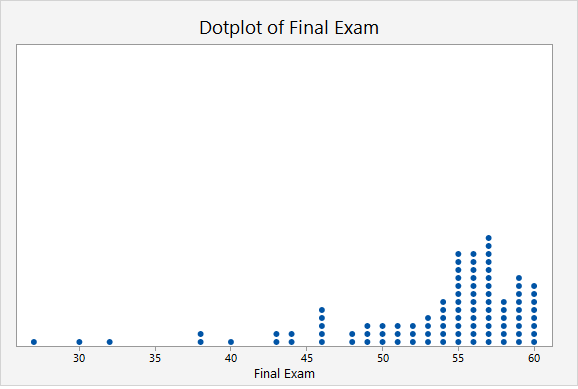# 2.2.9 - Percentiles

There are slightly different definitions of percentiles and different statistical software and textbooks may use different formulas. In this course, we will be using the definition from your textbook:

Percentile
Proportion of a distribution less than a given value.

## Example: Final Exam Scores Section

The dotplot below displays the final exam scores from a sample of 100 students. Estimate the 90th percentile.Given that there are 100 dots on this plot, the 90th percentile will fall around the 90th and 91st points. We can estimate the 90th percentile by counting down 10 from the top. The point that is 10th from the top is 59. Thus, the 90th percentile in this sample is a score of 59 points.